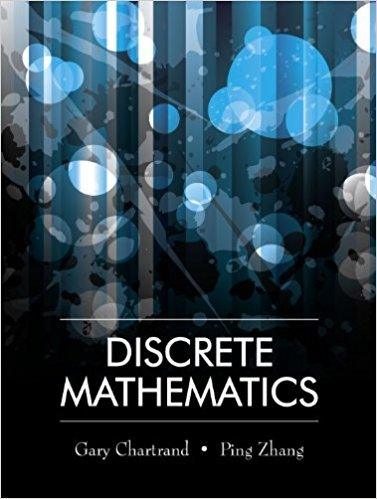×
×

# For two statements P and Q, use truth tables to verify the following. (a) (P Q) 6 ( P) (ISBN: 9781577667308 385

## Solution for problem 14 Chapter 1.2

Discrete Mathematics | 1st Edition

• Textbook Solutions
• 2901 Step-by-step solutions solved by professors and subject experts
• Get 24/7 help from StudySoup virtual teaching assistantsDiscrete Mathematics | 1st Edition

4 5 1 291 Reviews
23
2
Problem 14

For two statements P and Q, use truth tables to verify the following. (a) (P Q) 6 ( P) ( Q). (b) (P Q) 6 ( P) ( Q). 1

Step-by-Step Solution:
Step 1 of 3

L18 - 10 Now You Try It (NYTI): π 1. Find the equation of the tangent line to y =s n 3(x)cos(3 x)a t x = . 2 4 ▯ 2. Suppose that...

Step 2 of 3

Step 3 of 3

##### ISBN: 9781577667308

Unlock Textbook Solution